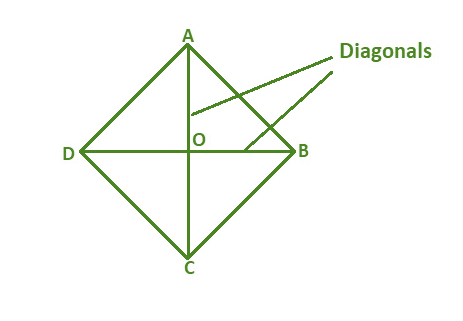GFG App
Open AppBrowser
Continue

# One of the diagonals of a rhombus of area 24 sq m is 6m what is the length of its other diagonal?

A rhombus is a diamond-shaped quadrilateral with equal sides but unequal angles of inclination between these two sides. It has four sides that are all the same length since it is a quadrilateral. It can be said that the rhombus is a specific type in a parallelogram, if all the sides of the parallelogram are to be equal, it will become a shape known as a rhombus.By using Diagonals Area of Rhombus:  = (d1 × d2)/2 sq. units.

Where d1 is the length of diagonal 1 and d2 is the length of diagonal 2.

### One of the diagonals of a rhombus of an area of 24 sq m is 6m what is the length of its other diagonal?

Solution:

To find the length of diagonal d2,

Area of Rhombus = 24 sq m

Length of diagonal d1 = 6 m

Therefore, Area of Rhombus = (d1 × d2)/2 sq. units

24  =  (6 × d2) / 2

24  =  3d2

d2 = 24/3

d2 = 8 m

So the length of diagonal d2 is 8 m.

### Sample Questions

Question 1:  Calculate the area of a rhombus (using diagonal) having diagonals equal to 5 cm and 4 cm.

Solution:

Given,

Length of diagonal 1 (d1) = 5 cm

Length of diagonal 2 (d2) = 4 cm

Now,

Area of Rhombus (A) = 1/2 d1 × d2

= 1/2  × 5 × 4

= 1/2 × 20

= 10 cm2

Question 2: Find the diagonal of a rhombus if its area is 100 cm2 and the length measure of the longest diagonal is 10 cm.

Solution:

Given: Area of rhombus = 100 cm2 and Diagonal d1 = 10 cm.

Hence, Area of the rhombus formula, A = (d1 × d2)/2 square units, we get

100 = (10 × d2)/2

100 = 10 d2 / 2

Or 5d2 = 100

d2 = 20

Therefore, the Length of another diagonal d2 is 20 cm.

Question 3: Calculate the area of a rhombus whose diagonals are of the lengths 15 cm and 4 cm?

Solution:

Given: Diagonal d1 = 15 cm

Diagonal d2 = 4 cm

Area of a rhombus, A = (d1 × d2) / 2

= (15 × 4) / 2

= 60/2

= 30 cm2

Hence, the area of a rhombus is 30 cm2.

Question 4:  Find the area of the rhombus where the side of the rhombus is 4 cm and one of the interior angles is 30°.

Solution:

Given,

Side length = 4 cm

Interior angle = 30°

Using Trigonometry , Area of rhombus = (side)2 Sin(30°)

= 42 × sin(30°)

= 16 × (1/2)

= 8 cm2

So the area of Rhombus is 8 cm2

Question 5: Find the perimeter of a rhombus whose side is 6 cm.

Solution:

Given side s = 6 cm

Therefore, Perimeter of Rhombus = 4 × s

So, Perimeter (P) = 4 × 6 cm

= 24 cm

Question 6: One of the diagonals of a rhombus of area 30 sq m is 6m what is the length of its other diagonal?

Solution:

To find the length of diagonal d2,

Area of Rhombus = 30 sq m

Length of diagonal d1 = 6 m

Therefore, Area of Rhombus  = (d1 × d2)/2 sq. units

30 = (6 × d2) / 2

30  =  3d2

d2 = 30/3

d2 = 10 m

So the length of diagonal d2 is 10 m.

Question 7: Find the height of the rhombus whose area is 200 m² and the perimeter is 100 m?

Solution:

Given, the perimeter of the rhombus = 120 m

So, side of rhombus = 100/4

= 25 m

We know that the area of Rhombus = b × h

Therefore the height is, 200 = 25 × h

h = 200 /25

h = 8

Therefore, the height of the rhombus is 8 m.

My Personal Notes arrow_drop_up